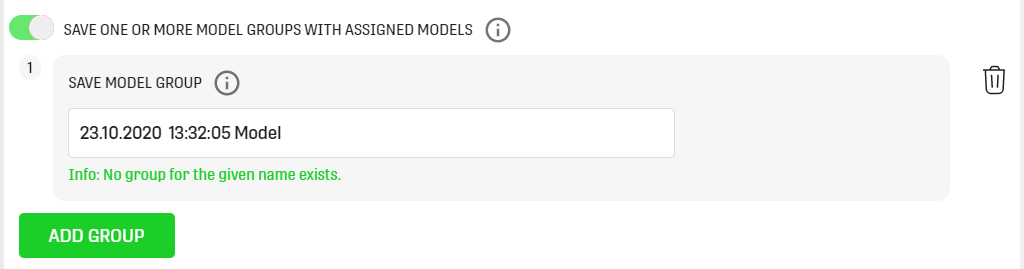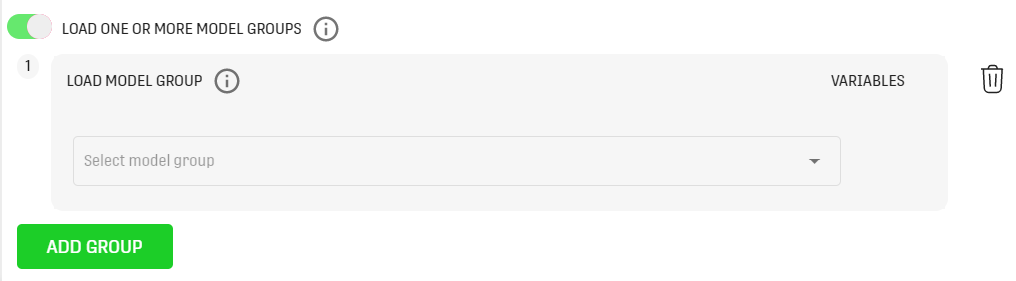# Motivation

With ONE DATA there are many ways to process data within a Workflow using different Processors. But sometimes, it is necessary or easier to use custom computation methods. A Python script, for instance, is a good way to customize the processing of data, due to the fact that Python is a highly flexible programming language with many open source libraries.

Since Python scripts are a very useful tool, it is possible to include them in Workflows using ONE DATA Python Processors. In this article, the focus will be on the Python Script Single Input Processor.

# Overview

The Python Script Single Input Processor takes a given input dataset and executes the specified script on it. Then the processed data can be passed as output to other Processors. There are already some useful libraries included that could be necessary to write scripts for data science tasks. The info button on the left corner of the input box provides further information on what packages are preinstalled.To interact with ONE DATA resources from within the Python script, for loading Models, accessing Variables or specifying the output of the Processor, it is necessary to use the ONE DATA Python Framework.

`For advanced usage of the ONE DATA Python Processors, it can be really helpful to have a deeper look into the framework. This article gives a small insight to it, but does not cover the framework in depth`

# Input

The Processor takes two valid datasets as input. The Python script which is used to process the input data can be inserted within the configuration:In the "Input Name In Script" textfields, it is possible to specify the names of the input datasets in the Python script. The default values are "input1" and "input2".

Then the inputs can be saved into variables, for example as Pandas Dataframe:

`dataset1 = od_input['input1'].get_as_pandas()dataset2 = od_input['input2'].get_as_pandas()`

or as 2D matrix:

`matrix = od_input['input1'].get_as_matrix()matrix = od_input['input2'].get_as_matrix()`

# Configuration

The Processor configuration gives some additional options on how the output data should be processed, along with some definitions for the script execution itself. It is also possible to load ONE DATA Python Models and use them in the script. All options are described more in-depth in the following sections.## Timeout for Script Execution

This is the time in seconds that ONE DATA waits for the script execution and the returning of the results of the script. The time starts when the Processor submits the Python script and the data to the Python Service of ONE DATA. If the timeout is exceeds, the calculation will be interrupted and a Processor execution error is thrown.

The default value is 300 seconds.

## Generate Empty Dataset Output

This option defines if the Processor should generate an empty dataset after the execution of the script. This can be very useful when the script is only used to generate a plot and no respective result dataset. This option can then be toggled to prevent a Processor execution error, since by default, it requires an output dataset.

## Manual TI

With this configuration option it is possible to specify what scale and representation type the columns of the output dataset have, in order to provide the correct type inference in ONE DATA.

Possible scale types: nominal, interval, ordinal, ratio. Further information on scale types can be found here.

Possible representation types: string, int, double, datetime, numeric

If it is not possible to convert the values of a column to the specified representation type, the Processor will take the type that fits best for its representation. If types still do not fit the purpose, it is recommended to use the Data type Conversion Processor.

## Load One or More ModelsThe first dropdown is used to select an existing Python Model from the current project. It's also possible to specify which version of the Model should be loaded.

With the "Open Model" button the view for the selected Model can be accessed directly from the Processor.

To use it in the script itself, a selected Model can be stored in a variable like so:

`model = od_models["model_name"]`

## Save One or More ModelsThis configuration option is used to save a generated Python Model to the project, or adds a new version to a existing one.

It has three options:

• Create New Model: Creates a new Model, with the name specified in the textbox. The name needs to be unique within a Domain.
• Add New Model Version: Adds a new version to an already existing Model which can be selected.
• Create Or Add Version: With this option the Processor either adds a new version to the given Model or creates a new one if the Model does not exist yet.

A Model can be saved using following script:

`od_output.add_model("my_model", model)`

`Note that, a Model needs to have a unique name within a Domain in ONE DATA.`

## Save One or More Model Groups With Assigned ModelsWith this option, you can save a Model Group created by Python within ONE DATA. All Model Groups added in the Python script must be configured in here, otherwise they will not be saved to the ONE DATA environment. To save a Model assigned to a Model Group stored in a variable Model under the name "my_model" and the Model Group name \"my_model_group\" use the following statement in the script:

`od_output.add_model("my_model", model, "my_model_group")`

`Note that, a Model Group needs to have a unique name within a Domain in ONE DATA.`

## Load One or More Model GroupsBy using this option, it is possible to load Model Groups for Python execution. Models of all loaded Model Groups will be accessible in the Python code in the dictionary: od_models

To load a Model named "my_model" and store it in a variable Model use the following statement:

`model = od_models["my_model"]`

# Output

The Python Script Dual Input Processor has several output types that can be defined within the script.

## Datasets

To pass a dataset as output to ONE DATA, the following method is used:

`od_output.add_data("output", dataset)`

Datasets can have the following formats:

• 2D matrix representation of data ( list of rows where each row is a list of column values) and list of column names
`from onelogic.odpf.common import ODDatasetfrom datetime import datetimedataset = ODDataset([[1, 2.0, "test", datetime.now()],                     [2, 3.0, "sample", datetime.now()]],                    ["int_col", "double_col", "str_col", "timestamp_col"])`

`from onelogic.odpf.common import ODDatasetfrom datetime import datetimefrom pandas import DataFramed = {'int_col': [1, 2], 'double_col': [2.0, 3.0], 'str_col': ['test', 'sample'],      'timestamp_col': [datetime.now(), datetime.now()]}dataset = ODDataset(DataFrame(data=d))`

Current restrictions:

• If content is passed as 2D matrix, column names must be specified and have the same size as each row
• Data types in columns must be supported

## Models

Like mentioned above in the configuration section, it is also possible to save Python Models to the project from within the script. This can be achieved like this:

`od_output.add_model("model_name", model_data)`

`Note that the "model_name" here has to exactly match the Model name specified in the Processor configuration.`

## Images

It is also possible to save plots and graphs generated within the script (for example using Pandas) as image to the "Image List" of the Processor. This can be done using the following method:

`od_output.add_image(image_name, image_type, image_data)`

where

• image_name is the name under which the image will be available in the Processor
• image_type is the type of the created image (either ImageType.PNG or ImageType.JPG)
• image_data is the image itself, either as byte array or a matplotlibs's Figure

## Logs

The Processor also has a log view, where logs produced with the "print()" statement can be viewed. It can be accessed by the clicking "Log content" on the left hand side toolbar.# Example

In this example, we have two input tables with simple numeric numbers. We compare each row of the dataset to the other and save the bigger number to the result list. Also at the beginning of the script, we print both datasets to the log.

## Script

`from onelogic.odpf import ImageTypeimport pandas as pd# od_input keys represent names of the input datasets set in OD Processor  dataset1 = od_input['left'].get_as_pandas()dataset2 = od_input['right'].get_as_pandas()result_list = []# print the datasetsprint(dataset1)print(dataset2)# iterate through the datasetsfor index, row in dataset1.iterrows():    left_row = row["Numbers"]    right_row = dataset2.iloc[index, :]['Numbers']        if right_row is not None and left_row is not None:        if (left_row <= right_row):            result_list.append(right_row)        else:            result_list.append(left_row)            # pass the output to ODresult_dataset = pd.DataFrame({'Bigger Number': result_list})od_output.add_data('output', result_dataset)`

## Input## Workflow## Configuration

For the left input dataset, the name "left" and for the right one, "right" is used.# Related Articles

Python Script Single Input Processor

Python Script Data Generator Processor

Hands on: Python Processors

ONE DATA Python Framework